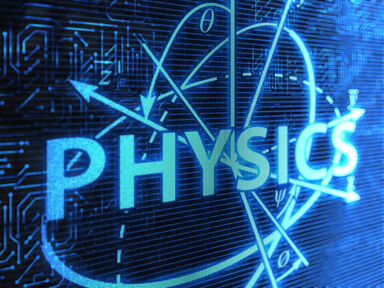# List of Basic Physics Terms

When you see the phrase physics terms, you might groan. However, once you dive into this area of science, you might find you like it. Not only does physics cover movement and energy, but it looks at the creation of the universe. Explore common physics terms and their meanings.black hole example of physics

## Physics Terms Covering Energy

Energy isn’t just a chemistry theory. Energy is important in physics too. However, when it comes to energy in physics, it’s all about motion.

• electrical energy - energy that comes from electrical charge
• electromagnetic energy - electrical and magnetic wave energy reflected and emitted from objects traveling through space
• gravitational energy - potential energy of an object in a higher position to the ground on earth (i.e. a book on a shelf has more gravitational energy than one on the floor)
• joule - unit used to measure electrical energy
• kinetic energy - energy in an object in motion
• mechanical energy - energy in an object due to its motion or position
• potential energy - energy stored in an object
• scalar - measurement of magnitude
• strong nuclear potential energy - strong energy of particles within the atom
• thermal energy - energy that heat generates
• weak nuclear potential energy - weak energy of particles within the atom

Physics is all about motion. You know that what goes up must come down. Now, it’s time to learn why.

• acceleration - how change in velocity is measured
• collision - when two objects collide
• displacement - the change in an object’s position
• force - the push or pull measurement of an object
• friction - motion resistance from objects rubbing against each other
• gravity - attraction force between objects, bodies, and masses in the universe
• inertia - a body's tendency to resist motion
• impulse - change in momentum
• power - rate or measurement at which work is done
• pressure - force pushing on an object
• momentum - how mass in motion is measured
• newton - how force is measured (think Isaac Newton)
• Newton’s First Law of Motion - objects stay at rest or in motion unless acted upon by a force
• Newton’s Second Law of Motion - the mass is proportional to the force it takes to accelerate it
• Newton’s Third Law of Motion - every action has an equal and opposite reaction
• simple machine - one of six types of basic devices to perform work
• vector - measurement of medium and direction of movement of magnitude
• speed - how fast something is moving; scalar quantity of magnitude of velocity vector
• velocity - rate and direction of motion measurement
• weight - measurement of mass of an object
• work - energy transfer measurement when an object moves

## Cool Physics Terms

Physics is an amazing field of study with some interesting and unique terms. Explore fun and cool physics terms you don’t see every day!

• absolute zero - theoretical lowest temperature
• alpha particle - helium nucleus radioactive substances emit
• acoustics - study of sound waves
• amplitude - wave height from the center of the wave
• big bang - theoretical explanation for beginning of the universe
• black hole - region of space that doesn’t allow anything, including light, to escape because of its powerful gravitational pull
• cosmic rays - particle traveling through space with a highly energetic atomic nucleus
• dark energy - gravity countering theoretical repulsive force which causes gravity to expand quickly
• entropy - measurement describing the randomness of a system
• fundamental forces - four fundamental forces that act between objects
• galaxy - the planets, stars, and other celestial bodies held together by one gravitational force
• nuclear fission - when an atom is split by impact with another particle or spontaneously
• principle of relativity - requires all physics equations to be in the same form
• quantum - the minimum energy needed for any object to be attracted to each other